# Octal To Decimal Conversion in C#

## Octal To Decimal Conversion in C# with Examples

In this article, I am going to discuss Octal to Decimal Conversion in C# with Examples. Please read our previous article, where we discussed Octal to Binary Conversion in C#. At the end of this article, you will understand, what are Decimal and Octal Number Systems is and how to convert an Octal Number into its equivalent Decimal Number using C#.

##### What is Octal Number System?

The octal numeral system or oct is the base-8 number system and uses the digits 0 to 7. Octal numerals can be made from binary numerals by grouping consecutive binary digits into groups of three (starting from the right). Example: (5303)8

##### What is Decimal Number System?

A number whose whole number part and Fractional part is separated by a decimal point or dot. This representation of the number is known as a decimal number. Decimal number system in mathematics, a positional numeral system employing 10 as the base requiring 10 different numerals, the digits 0,1,2,3,4,5,6,7,8,9. Example: 223.45

##### Octal to decimal Conversion example:

Convert (253)8 to decimal.

Solution: Given octal number is (253)8

First, we convert given into decimal and then add them.

(2*82) + (5*81) + (3*80) = 128 + 40+ 3 = 171

For better understanding, please have a look at the below diagram.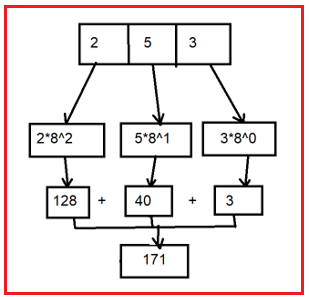##### Logic to Convert Octal to Decimal in C#

To find the conversion of octal to a decimal number, simply we need to follow the below steps.

1. First, we take the octal number.
2. Then we extract the digits of a given octal number starting from the rightmost digit and keep a variable dec_value
3. At the time of extracting digits from the octal number, multiply the digit with the proper base (Power of 8) and add it to the octal digit.
4. Then we store the number and we get the required decimal number.
###### In this program we are going to use the following data type
1. float: Floating-point numbers are used for decimal and exponential values. It is a primitive data type. It has 6 decimal digits of precision. Example: float volume = 20.93333
2. Int: Int data type is used to store the value of the integer in the program. It is a primitive data type. Example: Int a= 10;
3. long: long is a data type used in c# and other languages, long can store a single 64-bit signed integer. Example: long dec = 45798613;
##### Example: Octal to Decimal Number Conversion in C#

The following C# Program will convert an Octal number to its equivalent Decimal number.

```using System;
class OctalToDecimal
{
public static void Main()
{
int OctalNumber = 253;
Console.WriteLine(\$"Octal Number : {OctalNumber}");
int Decimal_Number = 0;
int BASE = 1;
int temp = OctalNumber;

while (temp > 0)
{
int last_digit = temp % 10;
temp /= 10;
Decimal_Number += last_digit * BASE;
BASE *= 8;
}
Console.WriteLine(\$"Decimal Number : {Decimal_Number}");
}
}
```
###### Output: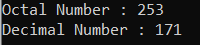##### Example: Taking input from the user

In the following C# program, we will take the octal number from the user and then convert the octal number to its equivalent decimal number and print it on the console.

```using System;
public class Octal_to_Decimal
{
public static void Main()
{
int n1, n5, p = 1, k, ch = 1;
int dec = 0, i = 1, j, d;
Console.WriteLine("Enter the Octal Number");
n5 = n1;

for (; n1 > 0; n1 = n1 / 10)
{
k = n1 % 10;
if (k >= 8)
{
ch = 0;
}
}

switch (ch)
{
case 0:
Console.Write("\nThe number is not an octal number. \n\n");
break;
case 1:
n1 = n5;
for (j = n1; j > 0; j = j / 10)
{
d = j % 10;
if (i == 1)
p = p * 1;
else
p = p * 8;

dec = dec + (d * p);
i++;
}
Console.Write("\nThe Octal Number : {0}\nThe equivalent Decimal  Number : {1} \n\n", n5, dec);
break;
}

}
}
```
###### Output1: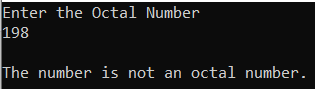###### Output2: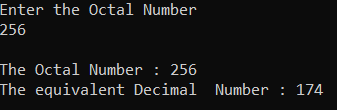##### C# Code (Using function)

In the below example, we have created one method to convert the octal number to its equivalent decimal number.

```using System;
class OctalToDecimal
{
public static void Main()
{
int OctalNumber = 253;
Console.WriteLine(\$"Octal Number : {OctalNumber}");
Console.WriteLine(\$"Decimal Number : {Octal_Decimal(OctalNumber)}");
}

private static int Octal_Decimal(int OctalNumber)
{
int Decimal_Number = 0;
int BASE = 1;
int temp = OctalNumber;

while (temp > 0)
{
int last_digit = temp % 10;
temp /= 10;
Decimal_Number += last_digit * BASE;
BASE *= 8;
}

return Decimal_Number;
}
}
```
###### Output: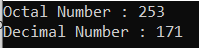Here, in this article, I try to explain how to convert an octal number to its equivalent hexadecimal number in C# with Examples and I hope you enjoy this Octal to Decimal Conversion in C# article.